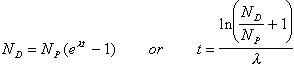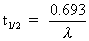# EARTH SCIENCE LAB Radiometric Dating

### Radiometric Dating - Graphical Method

The purpose of this portion of this exercise is to practice determining radiometric ages using graphical techniques and mathematical techniques. Consult your lab manual and materials for details.

Complete columns 1 and 2 in the table below. For example, after one half-life 0.5 of the original parent isotope remains, 0.5 of the sample is now the daughter isotope. After two half-lives 0.25 of the original parent isotope remains, 0.75 of the sample is now the daughter isotope.

Complete column 3. Divide the value in column 2 by the value in column 1. Enter the appropriate value in the space provided. Once all values have been entered, check you answers by clicking on Grade Daughter/Parent Ratio. (Note: Only column 3 will be graded)

 Half-Lives Elapsed 1. Parent Isotope 2. Daughter Isotope 3. Daughter/Parent Ratio 0 1 0.5 0.5 1 2 0.25 0.75 3 4 5

For samples 1, 2 and 3 determine the ND/NP ratio in the table below (calculate and enter each value to 2 decimal places - 3.454 is entered as 3.45; 12 is entered as 12.00; All values less than 1 must have a "0" in front of the decimal - 0.35, 0.50, etc.). NP is the number of parent atoms. ND is the number of daughter atoms.

Using the graph, determine the number of half-lives elapsed for each sample. (estimate each value to 1 decimal place - 1.5, 6.3, etc.)

If the half-life is 5,730 years, determine the age of the sample. (enter all values without commas - example 5,678 would be entered as 5678; calculate all values to the nearest whole number)

 Sample NP ND ND / NP Half-lives Elapsed Age in Years 1 736 1472 2 918 6426 3 528 12144### Radiometric Dating - Mathematical Method

Mathematical calculation of radiometric dating involves the use of a simple equation. The age of a mineral is determined from the number of parent and daughter isotopes it contains. The greater the number of daughter isotopes, the older the mineral. Ages can be determined using the equation:in which NP is the number of parent atoms and ND is the number of daughter atoms. e is the natural logarithm base, λ is the decay constant and t is time in years.

For samples 1, 2 and 3 determine the ND/NP ratio in the table below (calculate each value to 2 decimal places). NP is the number of parent atoms. ND is the number of daughter atoms.

If the decay constant (λ) for the radioactive decay of 40K is λ = 5.543 x 10-10/year, determine the age of the samples in the table below. (Round each answer to the nearest million years - example 873,732,221 years would be entered as 874000000; 9,845,123,004 years would be entered as 9845000000)

The half-life (t1/2) of 40K can be determined from the decay constant:What is the half-life of 40K? (Round the answer to the nearest million years - as shown above)

Using the half-life calculated above, complete the Half-lives Elapsed column in the table. (calculate each value to 2 decimal places)

 Sample NP ND ND / NP Age in Years Half-lives Elapsed 1 2486 2260 2 9134 7125 3 760 1254